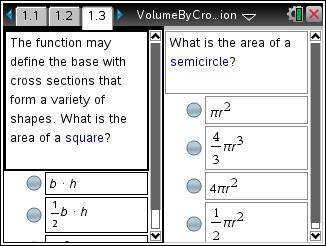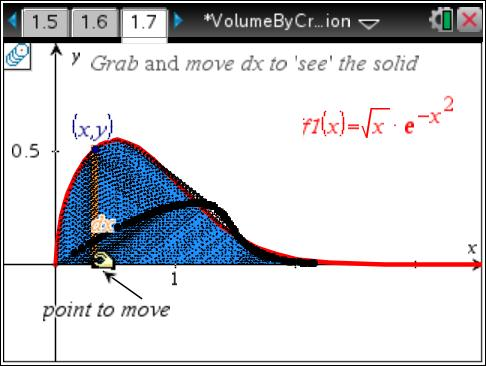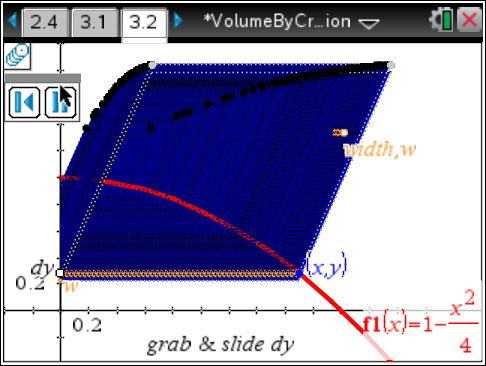Activities

••• Subject Area

• Math: Calculus: Applications of Integral, Antiderivatives and Definite Integrals
• Math: Calculus: Antiderivatives and Slope Fields

• Author9-12

15 Minutes

• Device
•TI-Nspire™ CX/CX II
•TI-Nspire™ CX CAS/CX II CAS
• TI-Nspire™ Navigator™
• TI-Nspire™
• TI-Nspire™ CAS
• Software

TI-Nspire™
TI-Nspire™ CAS

3.2

• Report an Issue

Volume by Cross SectionsActivity Overview

Students will be introduced to the concept of finding the volume of a solid formed by cross sections of a function that form certain shapes.

Key Steps

•Students will be introduced to the concept of finding the volume of a solid formed by cross sections of a function that form certain shapes. Since volume is the area of the base times the height and V = Area∙dx, student review areas of various shapes like squares, semicircles and equilateral triangles using self-check questions.

•Geometry Trace is used to help students get a 3D visual of the volume under consideration.

•Students will practice what they learn with exam-like multiple choice questions for homework or as an extension.